# Plücker coordinates

The coordinates of a straight line in three-dimensional space, the six numbers, and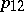, of which the first three are the coordinates of the direction vectorfor the straight lineand the second three are the moments of this vector about the origin. Let the linepass through the pointsandwith projective coordinates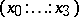and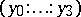, respectively; the Plücker coordinates for this line are the numbersThe Plücker coordinates are used in line geometry. They were first considered by J. Plücker (1869). Sometimes, instead of the Plücker coordinates one uses the Klein coordinates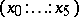, which are related to the Plücker ones as follows: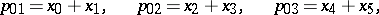More generally, one naturally considers the Plücker coordinates as coordinates of a-dimensional vector subspace of an-dimensional vector space. Then they are understood as the set of numbers equal to-subdeterminants of the-matrixwith as columns,, the coordinate columns (in some basis for) of the basis vectors of a subspace. If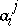are the components of a column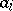,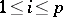, then the Plücker coordinates (or Grassmann coordinates) are the numbers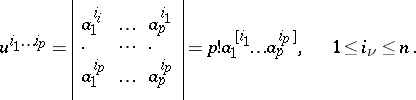The Plücker coordinates are anti-symmetric in all indices. The number of significant Plücker coordinates is.

When the basis ofis changed and the basis foris fixed, the Plücker coordinates are all multiplied by the same non-zero number. When the basis ofis changed and the basis foris fixed, the Plücker coordinates transform as the components of a contravariant tensor of valency(see Poly-vector). Two subspaces coincide if and only if their Plücker coordinates, calculated in the same basis for, differ only by a non-zero factor.

A vectorbelongs to a subspaceif the linear equations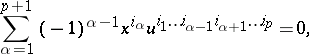with coefficients that are the Plücker coordinates for, are fulfilled. In these equationsare all possible sets of numbers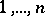.

Relating the Plücker and Klein coordinates as above, the Plücker identity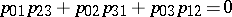becomes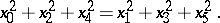The Plücker coordinates of-dimensional subspacesof an-dimensional space(over any field) define an imbedding of the Grassmann variety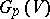into-dimensional projective space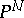with. As a subvariety of,is given by quadratic relations, the Plücker relations, which look as follows:i.e. take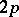indices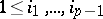;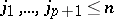and write down the relation above, using thatif two of the's are equal. If,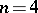, there is just one relation: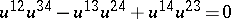.